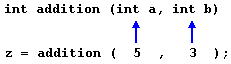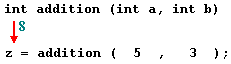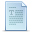# Functions (I)

Using functions we can structure our programs in a more modular way, accessing all the potential that structured programming can offer to us in C++.

A function is a group of statements that is executed when it is called from some point of the program. The following is its format:

type name ( parameter1, parameter2, ...) { statements }

where:
• type is the data type specifier of the data returned by the function.
• name is the identifier by which it will be possible to call the function.
• parameters (as many as needed): Each parameter consists of a data type specifier followed by an identifier, like any regular variable declaration (for example: int x) and which acts within the function as a regular local variable. They allow to pass arguments to the function when it is called. The different parameters are separated by commas.
• statements is the function's body. It is a block of statements surrounded by braces { }.

Here you have the first function example:

 ``123456789101112131415161718`` ``````// function example #include using namespace std; int addition (int a, int b) { int r; r=a+b; return (r); } int main () { int z; z = addition (5,3); cout << "The result is " << z; return 0; }`````` `The result is 8`

In order to examine this code, first of all remember something said at the beginning of this tutorial: a C++ program always begins its execution by the main function. So we will begin there.

We can see how the main function begins by declaring the variable z of type int. Right after that, we see a call to a function called addition. Paying attention we will be able to see the similarity between the structure of the call to the function and the declaration of the function itself some code lines above:The parameters and arguments have a clear correspondence. Within the main function we called to addition passing two values: 5 and 3, that correspond to the int a and int b parameters declared for function addition.

At the point at which the function is called from within main, the control is lost by main and passed to function addition. The value of both arguments passed in the call (5 and 3) are copied to the local variables int a and int b within the function.

Function addition declares another local variable (int r), and by means of the expression r=a+b, it assigns to r the result of a plus b. Because the actual parameters passed for a and b are 5 and 3 respectively, the result is 8.

The following line of code:

 `` `` ``return (r);``

finalizes function addition, and returns the control back to the function that called it in the first place (in this case, main). At this moment the program follows its regular course from the same point at which it was interrupted by the call to addition. But additionally, because the return statement in function addition specified a value: the content of variable r (return (r);), which at that moment had a value of 8. This value becomes the value of evaluating the function call.So being the value returned by a function the value given to the function call itself when it is evaluated, the variable z will be set to the value returned by addition (5, 3), that is 8. To explain it another way, you can imagine that the call to a function (addition (5,3)) is literally replaced by the value it returns (8).

The following line of code in main is:

 `` `` ``cout << "The result is " << z;``

That, as you may already expect, produces the printing of the result on the screen.

#### Scope of variables

The scope of variables declared within a function or any other inner block is only their own function or their own block and cannot be used outside of them. For example, in the previous example it would have been impossible to use the variables a, b or r directly in function main since they were variables local to function addition. Also, it would have been impossible to use the variable z directly within function addition, since this was a variable local to the function main.Therefore, the scope of local variables is limited to the same block level in which they are declared. Nevertheless, we also have the possibility to declare global variables; These are visible from any point of the code, inside and outside all functions. In order to declare global variables you simply have to declare the variable outside any function or block; that means, directly in the body of the program.

And here is another example about functions:

 ``12345678910111213141516171819202122`` ``````// function example #include using namespace std; int subtraction (int a, int b) { int r; r=a-b; return (r); } int main () { int x=5, y=3, z; z = subtraction (7,2); cout << "The first result is " << z << '\n'; cout << "The second result is " << subtraction (7,2) << '\n'; cout << "The third result is " << subtraction (x,y) << '\n'; z= 4 + subtraction (x,y); cout << "The fourth result is " << z << '\n'; return 0; }`````` ```The first result is 5 The second result is 5 The third result is 2 The fourth result is 6```

In this case we have created a function called subtraction. The only thing that this function does is to subtract both passed parameters and to return the result.

Nevertheless, if we examine function main we will see that we have made several calls to function subtraction. We have used some different calling methods so that you see other ways or moments when a function can be called.

In order to fully understand these examples you must consider once again that a call to a function could be replaced by the value that the function call itself is going to return. For example, the first case (that you should already know because it is the same pattern that we have used in previous examples):

 ``12`` ``````z = subtraction (7,2); cout << "The first result is " << z;``````

If we replace the function call by the value it returns (i.e., 5), we would have:

 ``12`` ``````z = 5; cout << "The first result is " << z;``````

As well as

 `` `` ``cout << "The second result is " << subtraction (7,2);``

has the same result as the previous call, but in this case we made the call to subtraction directly as an insertion parameter for cout. Simply consider that the result is the same as if we had written:

 `` `` ``cout << "The second result is " << 5;``

since 5 is the value returned by subtraction (7,2).

In the case of:

 `` `` ``cout << "The third result is " << subtraction (x,y);``

The only new thing that we introduced is that the parameters of subtraction are variables instead of constants. That is perfectly valid. In this case the values passed to function subtraction are the values of x and y, that are 5 and 3 respectively, giving 2 as result.

The fourth case is more of the same. Simply note that instead of:

 `` `` ``z = 4 + subtraction (x,y);``

we could have written:

 `` `` ``z = subtraction (x,y) + 4;``

with exactly the same result. I have switched places so you can see that the semicolon sign (;) goes at the end of the whole statement. It does not necessarily have to go right after the function call. The explanation might be once again that you imagine that a function can be replaced by its returned value:

 ``12`` ``````z = 4 + 2; z = 2 + 4;``````

### Functions with no type. The use of void.

If you remember the syntax of a function declaration:

type name ( argument1, argument2 ...) statement

you will see that the declaration begins with a type, that is the type of the function itself (i.e., the type of the datum that will be returned by the function with the return statement). But what if we want to return no value?

Imagine that we want to make a function just to show a message on the screen. We do not need it to return any value. In this case we should use the void type specifier for the function. This is a special specifier that indicates absence of type.

 ``1234567891011121314`` ``````// void function example #include using namespace std; void printmessage () { cout << "I'm a function!"; } int main () { printmessage (); return 0; }`````` `I'm a function!`

void can also be used in the function's parameter list to explicitly specify that we want the function to take no actual parameters when it is called. For example, function printmessage could have been declared as:

 ``1234`` ``````void printmessage (void) { cout << "I'm a function!"; }``````

Although it is optional to specify void in the parameter list. In C++, a parameter list can simply be left blank if we want a function with no parameters.

What you must always remember is that the format for calling a function includes specifying its name and enclosing its parameters between parentheses. The non-existence of parameters does not exempt us from the obligation to write the parentheses. For that reason the call to printmessage is:

 `` `` ``printmessage ();``

The parentheses clearly indicate that this is a call to a function and not the name of a variable or some other C++ statement. The following call would have been incorrect:

 `` `` ``printmessage;``Previous:Simple control structuresIndexNext:Functions (II)